Next: Basic Circuit Configurations Up: Bipolar Junction Transistors Previous: Bipolar Junction Transistors

## Transistor Operation (NPN)

If the collector, emitter, and base of an NPN transistor are shorted together as shown in figure 5.2a, the diffusion process described earlier for diodes results in the formation of two depletion regions that surround the base as shown. The diffusion of negative carriers into the base and positive carriers out of the base results in a relative electric potential as shown in figure 5.2b.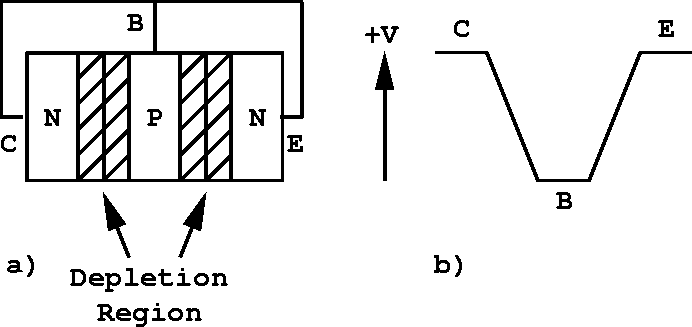Figure 5.2:  a) NPN transistor with collector, base and emitter shorted together, and b) voltage levels developed within the shorted semiconductor.

When the transistor is biased for normal operation as in figure 5.3a, the base terminal is slightly positive with respect to the emitter (about 0.6 V for silicon), and the collector is positive by several volts. When properly biased, the transistor acts to make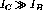. The depletion region at the reverse-biased base-collector junction grows and is able to support the increased electric potential change indicated in the figure 5.3b.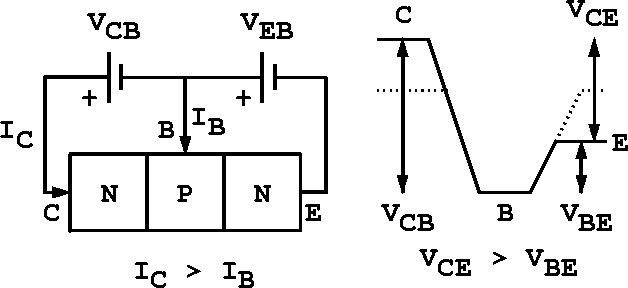Figure 5.3:  a) NPN transistor biased for operation and b) voltage levels developed within the biased semiconductor.

For a typical transistor, 95% to 99% of the charge carriers from the emitter make it to the collector and constitute almost all the collector current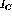.is slightly less than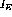and we may write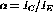, where from above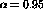to 0.99.

The behaviour of a transistor can be summarized by the characteristic curves shown in figure 5.4. Each curve starts from zero in a nonlinear fashion, rises smoothly, then rounds a knee to enter a region of essentially constant. This flat region corresponds to the condition where the depletion region at the base-emitter junction has essentially disappeared. To be useful as a linear amplifier, the transistor must be operated exclusively in the flat region, where the collector current is determined by the base current.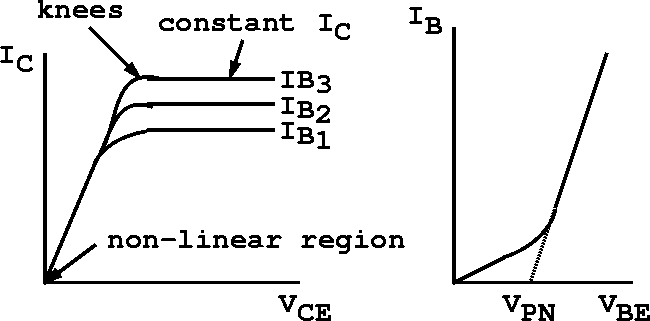Figure 5.4:  Characteristic curves of an NPN transistor.

A small current flow into the base controls a much larger current flow into the collector. We can write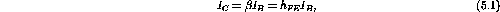whereis the DC current gain and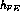is called the static forward-current transfer ratio. From the previous definition ofand the conservation of charge,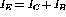, we have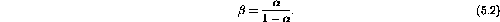For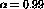we have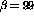and the transistor is a current amplifying device.Next: Basic Circuit Configurations Up: Bipolar Junction Transistors Previous: Bipolar Junction Transistors

Doug Gingrich
Tue Jul 13 16:55:15 EDT 1999